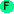# Evaluation

## Evaluation

Arc provides several syntax innovations beyond Scheme.

## Square-bracket anonymous functions

Anonymous functions on one variable can be created with square brackets; the underscore `_` is the variable. For example, `[+ 1 _]` is equivalent to `(fn (_) (+ 1 _))`:
```arc> (map [+ 1 _] '(10 20 30))
(11 21 31)
```

## Special syntax

The "special syntax" characters are `:`, `~`, `.`, and `!`. The `~` character provides Boolean complement of a procedure; `~a` is equivalent to `(complement a)`
```arc> (~odd 3)
nil
arc> (~even 3)
t
```
The `:` character implements function composition; `a:b` is equivalent to `(compose a b)` and applies `a` to the result of applying `b`:
```arc> (odd:sqrt 4)
nil
arc> (odd:sqrt 9)
t
```
The syntax `a.b` is equivalent to `(a b)`. It is intended for structure access:
```arc> (= x '(a b c d))
(a b c d)
arc> x.2
c
```
The syntax `a!b` is equivalent to `(a 'b)`. It is intended for structure access where quoting is needed:
```arc> (= tb (obj a 10 b 20 c 30))
#hash((c . 30) (a . 10) (b . 20))
arc> tb!a
10
```eval expression Evaluates the expression. ```>(eval '(+ 1 2)) 3 ```apply fn arglist Applies the function to the arguments. ```>(apply + '(1 2)) 3 ```ssexpand symbol Expands special syntax (: ! . or ~). The : character indicates composition. The ~ indicates complementing. The . applies the first value to the remainder. The ! is like . except the arguments are quoted. ```>(ssexpand 'x:~y:z) (compose x (complement y) z) ``` ```>(ssexpand '+.1.2) ((+ 1) 2) ``` ```>(ssexpand '+!1!2) ((+ (quote 1)) (quote 2)) ``` ```>(ssexpand 'cons!a!b) ((cons (quote a)) (quote b)) ```ssyntax symbol Tests if the symbol contains special syntax (: ! . or ~). ```>(ssyntax 'x:y) t ```compose [function] ... Composes the functions. ```>((compose sqrt:len) "abcd") 2 ```complement function Creates a procedure that is the complement of `function`. ```>(map (complement odd) '(1 2 3 4 5)) (nil t nil t nil) ```idfn x Identity function; returns x.